Test: Quantitative Techniques- 8

# Test: Quantitative Techniques- 8

Test Description

## 20 Questions MCQ Test | Test: Quantitative Techniques- 8

Test: Quantitative Techniques- 8 for Banking Exams 2023 is part of Banking Exams preparation. The Test: Quantitative Techniques- 8 questions and answers have been prepared according to the Banking Exams exam syllabus.The Test: Quantitative Techniques- 8 MCQs are made for Banking Exams 2023 Exam. Find important definitions, questions, notes, meanings, examples, exercises, MCQs and online tests for Test: Quantitative Techniques- 8 below.
Solutions of Test: Quantitative Techniques- 8 questions in English are available as part of our course for Banking Exams & Test: Quantitative Techniques- 8 solutions in Hindi for Banking Exams course. Download more important topics, notes, lectures and mock test series for Banking Exams Exam by signing up for free. Attempt Test: Quantitative Techniques- 8 | 20 questions in 30 minutes | Mock test for Banking Exams preparation | Free important questions MCQ to study for Banking Exams Exam | Download free PDF with solutions
 1 Crore+ students have signed up on EduRev. Have you?
Test: Quantitative Techniques- 8 - Question 1

### A fan is listed at Rs1500 and A discount of 20% is offered at the listed price. What additional discount must be offered to bring net price to Rs 1104?

Detailed Solution for Test: Quantitative Techniques- 8 - Question 1

M.P → Rs 1500
After 1st discount → 80% of 1500 = 1200
For S.P to be 1104, additional RS 96 discount required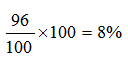Test: Quantitative Techniques- 8 - Question 2

### The least value of x for which the expression, x2+x+17, will not give a prime number?

Test: Quantitative Techniques- 8 - Question 3

### A thief is spotted by a policeman, from a distance of 200m.the policeman and thief start running at the speed of 20kmph and 16kmph. How far the police man will have to run so as to catch the thief

Detailed Solution for Test: Quantitative Techniques- 8 - Question 3

Net advantage = 4 Km is 1 hr.
i.e. 4000 m in 1 hr.
So, for 200 m advantage time required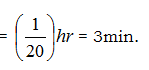Now, Policeman runs 20 km in 1 hr.
So in 3 min he will run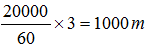Test: Quantitative Techniques- 8 - Question 4

If the radius of a circle is increased by 50% then the area is increased by?

Detailed Solution for Test: Quantitative Techniques- 8 - Question 4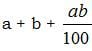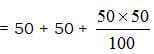= 100 + 25 = 125%

Test: Quantitative Techniques- 8 - Question 5

If 0.06% of a number is 84, then 30% of that number is?

Detailed Solution for Test: Quantitative Techniques- 8 - Question 5

Let the no. be x
x*6/100 =84
x=84*100/6
=14*100
=1400
30% of 1400 is
1400*30/100
14*30
=420

Test: Quantitative Techniques- 8 - Question 6

A sum was divided among P, Q, R. R got double than P, who got double than Q. If the difference between the shares of Q and R is Rs 3675, then the sum was?

Detailed Solution for Test: Quantitative Techniques- 8 - Question 6

R : P  2 : 1   R : P : Q  Diff (R,Q)
P : Q  2 : 1 →   3675
3 ≡ 3675
1 ≡ 1225
Sum > ≡ 1225 x 7 = 8575

Test: Quantitative Techniques- 8 - Question 7

One side of rectangular field is 15m and one of the diagonal is 17m. Find the area of the field

Detailed Solution for Test: Quantitative Techniques- 8 - Question 7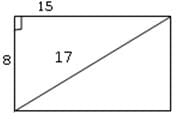Area = 15 x 8 = 120 m2

Test: Quantitative Techniques- 8 - Question 8

The denominator of a fraction is greater than it’s numerator by 11. If 8 is added to both it’s numerator and denominator, then it becomes 3/4 the fraction is?

Test: Quantitative Techniques- 8 - Question 9

The difference of two number is 11 and one-fifth of their sum is 9 find the numbers?

Detailed Solution for Test: Quantitative Techniques- 8 - Question 9

28 + 17 = 45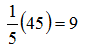Test: Quantitative Techniques- 8 - Question 10

The average of five consecutive even numbers A, B, C, D and E is 96. What is the product of A and E?

Detailed Solution for Test: Quantitative Techniques- 8 - Question 10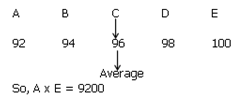Test: Quantitative Techniques- 8 - Question 11

The difference between the age of two person is 8 years. 12 Years ago, the elder one was twice the age of younger one. What is the ratio of their present ages?

Detailed Solution for Test: Quantitative Techniques- 8 - Question 11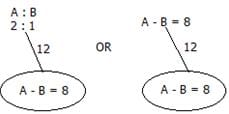So, 1 ≡ 8yrs
2 ≡ 16 yrs
So ratio of their present ages = 20 : 28 = 5 : 7

Test: Quantitative Techniques- 8 - Question 12

40% of the greater number is equal to 60% of the smaller. If their sum is 150 then the greater no. is?

Detailed Solution for Test: Quantitative Techniques- 8 - Question 12

40% of G = 60% S,   Now G : S = 3 : 2
3 + 2 ≡ 150   so 5 ≡ 150
1 ≡ 30
So 3 ≡ 90

Test: Quantitative Techniques- 8 - Question 13

Successive discount of 10% and 20% is equivalent to single discount of ?

Detailed Solution for Test: Quantitative Techniques- 8 - Question 13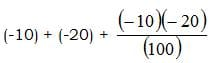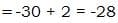Test: Quantitative Techniques- 8 - Question 14

Two metals contain zinc and copper in the ratio 2:1 and 4:1 respectively. In what ratio these two must be mixed to get a new metal containing zinc and copper in the ratio 3:1?

Detailed Solution for Test: Quantitative Techniques- 8 - Question 14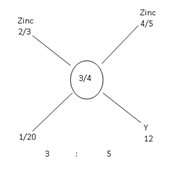Test: Quantitative Techniques- 8 - Question 15

Alok started a business with a sum of rs.50000. After a year, he again invested Rs 30000 and made Sudhir his partner who invested Rs 70000. At the end of the 3 years, total profit was Rs 87500. What is the share of Sudhir?

Detailed Solution for Test: Quantitative Techniques- 8 - Question 15

A : S
(50,000 x 1) (70,000 x 2) (80,000 x 2)
2,10,000 1,40,000 Sum
3 : 2 → 87500
5 ≡ 87500
1 ≡ 17500
2 ≡ 35000

Test: Quantitative Techniques- 8 - Question 16

Two trains 128 meters and 122 meters long are running towards each other on parallel lines at 48 kmph and 42kmph respectively. In what time will they clear off each other from the moment they meet?

Detailed Solution for Test: Quantitative Techniques- 8 - Question 16
D = 120 + 80 = 200 M
S = 72 km/h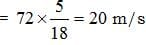T = 20/20 = 1 sec
Test: Quantitative Techniques- 8 - Question 17

Pipe A can fill the tank in 3hrs and pipe B can fill the tank in 4hrs. An outlet pipe C can empty the tank in 6 hrs. If all pipes are kept open simultaneously, in how many hours will the tank be half full?

Detailed Solution for Test: Quantitative Techniques- 8 - Question 17

Tank capacity = 12 L
A + B → 7L in 1 min
C → -2L in 1 min
So Net result = 5L in 1min
So, time taken = 12/5

Test: Quantitative Techniques- 8 - Question 18

In how many years a certain sum doubles itself at 4% per annum simple interest ?

Detailed Solution for Test: Quantitative Techniques- 8 - Question 18

P = 100, S.I = 100, R = 4%, t = ??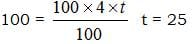Test: Quantitative Techniques- 8 - Question 19

I can row 40km downstream and 28km upstream taking 10hours each time-what is the speed of current?

Detailed Solution for Test: Quantitative Techniques- 8 - Question 19

Speed downstream = 40/10 = 4hrs
Speed upstream = 28/10 = 2.8 hrs
Spees upstream  = 4-2.8/2
= 1.2/2 = 0.6

Test: Quantitative Techniques- 8 - Question 20

Three numbers are in the ratio 1:2:3 and their HCF is 12.the numbers are?

Detailed Solution for Test: Quantitative Techniques- 8 - Question 20

The correct option is D.
The HCF in x, 2x and 3x is x because 1, 2, 3 are prime. Hence, x = 12; then the other numbers are 24 and 36.

Information about Test: Quantitative Techniques- 8 Page
In this test you can find the Exam questions for Test: Quantitative Techniques- 8 solved & explained in the simplest way possible. Besides giving Questions and answers for Test: Quantitative Techniques- 8, EduRev gives you an ample number of Online tests for practice(Scan QR code)